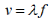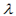# Since v = λ f, the speed v of a wave depends on its frequency f. True/False?

Since v = λ f, the speed v of a wave depends on its frequency f.
True/FalseHere,is the wavelength, which is the distance across the wave repeats and (f) is the frequency, where the number of waves are passed at a given point.